# Python Data Type

## Introduction

Type represents the kind of value and determines how the value can be used. All data values in Python are encapsulated in relevant object classes. Everything in Python is an object and every object has an identity, a type, and a value. Like another object-oriented language such as Java or C++, there are several data types which are built into Python. Extension modules which are written in C, Java, or other languages can define additional types.

To determine a variable's type in Python you can use the type() function. The value of some objects can be changed. Objects whose value can be changed are called mutable and objects whose value is unchangeable (once they are created) are called immutable. Here are the details of Python data types

## Numbers

Numbers are created by numeric literals. Numeric objects are immutable, which means when an object is created its value cannot be changed.

Python has three distinct numeric types: integers, floating point numbers, and complex numbers. Integers represent negative and positive integers without fractional parts whereas floating point numbers represents negative and positive numbers with fractional parts. In addition, Booleans are a subtype of plain integers. See the following statements in Python shell.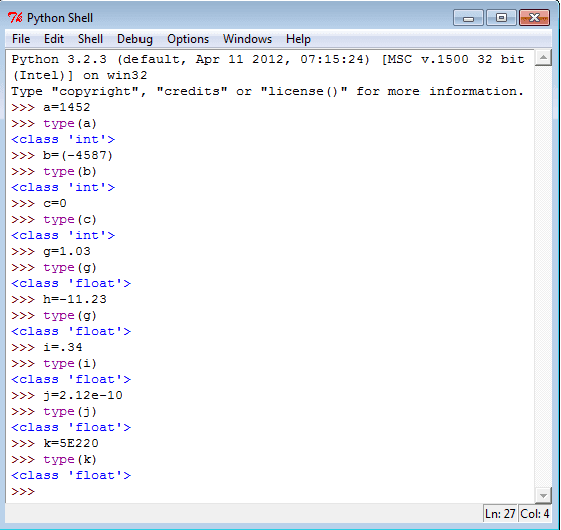Floats and Integers

``````>>> x = 8
>>> y = 7
>>> x+y
>>> x-y
>>> x/y
>>> x*y
``````

Output:

```15
1
1.1428571428571428
56
```

Exponent

``````>>> 4**3
>>> 3**4
``````

Output:

```64
81
```

inter division

``````>>> 12/3
>>> 64//4
>>> 15//3
``````

Output:

```4.0
16
5
```

Remainder

``````>>> 15 % 4
``````

Output:

```3
```

Mathematically, a complex number (generally used in engineering) is a number of the form A+Bi where i is the imaginary number. Complex numbers have a real and imaginary part. Python supports complex numbers either by specifying the number in (real + imagJ) or (real + imagj) form or using a built-in method complex(x, y). See the following statements in Python Shell.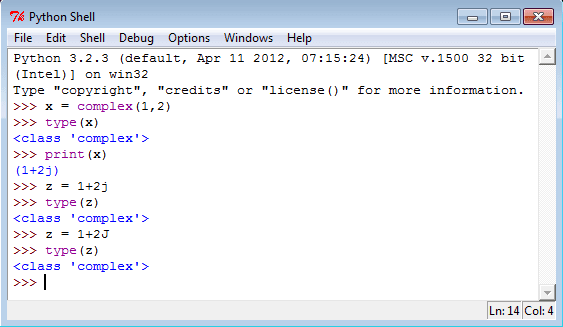## Self-assignment

``````>>> count = 0
>>> count +=2
>>> count
``````

Output:

```2
```
``````>>> count -=2
>>> count
``````

Output:

```0
```
``````>>> count +=2
>>> count *=4
>>> count
``````

Output:

```8
```
``````>>> count **=4
>>> count
``````

Output:

```4096
```
``````>>> count /=4
>>> count
``````

Output:

```1024.0
```
``````>>> count//=4
>>> count
``````

Output:

```256.0
```
``````>>> 15.0 //2
``````

Output:

```7.0
```

Order of operations

``````>>> (3+12) / 3
>>> 15 / (3 + 2)
``````

Output:

```5.0
3.0
```

## Boolean (bool)

The simplest build-in type in Python is the bool type, it represents the truth values False and True. See the following statements in Python shell.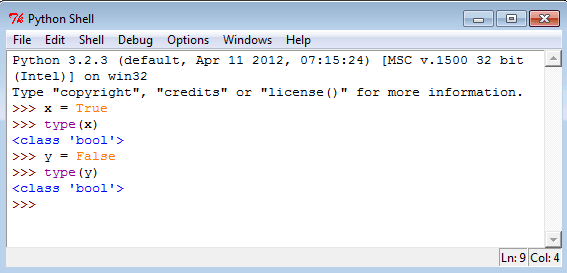## Strings

In Python, a string type object is a sequence (left-to- right order) of characters. Strings start and end with single or double quotes Python strings are immutable. Single and double quoted strings are same and you can use a single quote within a string when it is surrounded by double quote and vice versa. Declaring a string is simple, see the following statements.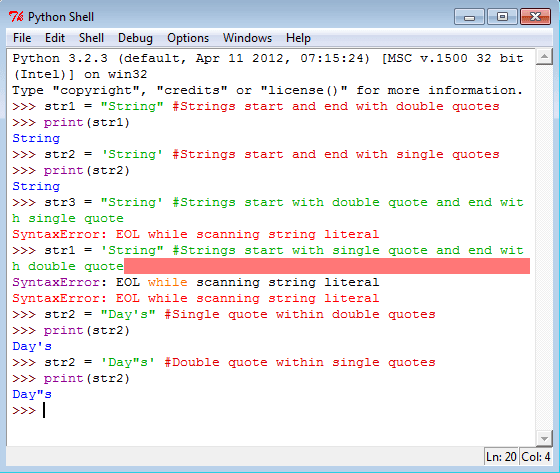## Special characters in strings

The backslash (\) character is used to introduce a special character. See the following table.

 Escape sequence Meaning \n Newline \t Horizontal Tab \\ Backslash \' Single Quote \" Double Quote

See the following statements on special characters.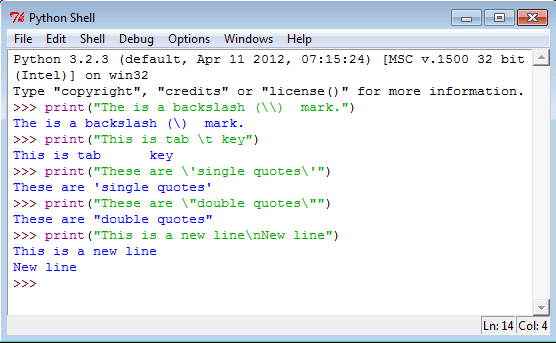## String indices and accessing string elements

Strings are arrays of characters and elements of an array can be accessed using indexing. Indices start with 0 from left side and -1 when starting from right side.

string1 ="PYTHON TUTORIAL"

 Character P Y T H O N T U T O R I A L Index (from left) 0 1 2 3 4 5 6 7 8 9 10 11 12 13 14 Index (from right) -15 -14 -13 -12 -11 -10 -9 -8 -7 -6 -5 -4 -3 -2 -1

See the following statements to access single character from various positions.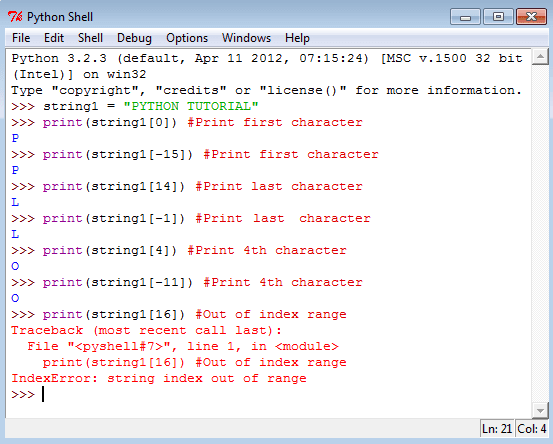## Strings are immutable

Strings are immutable character sets. Once a string is generated, you can not change any character within the string. See the following statements.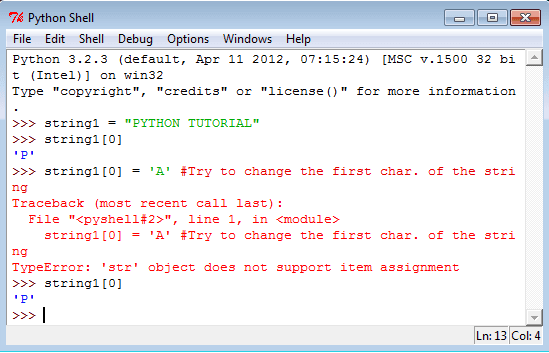## 'in' operator in Strings

The 'in' operator is used to check whether a character or a substring is present in a string or not. The expression returns a Boolean value. See the following statements.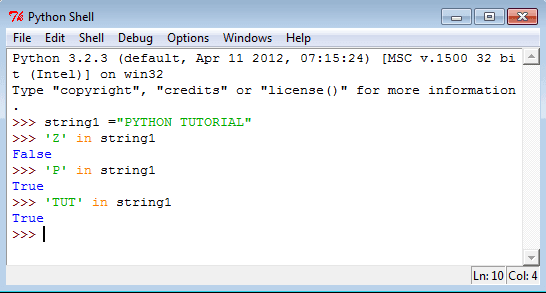## String Slicing

To cut a substring from a string is called string slicing. Here two indices are used separated by a colon (:). A slice 3:7 means indices characters of 3rd, 4th, 5th and 6th positions. The second integer index i.e. 7 is not included. You can use negative indices for slicing. See the following statements.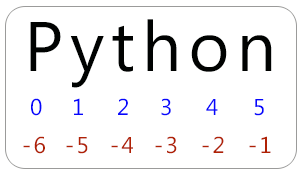## Conversion

``````>>> float("4.5")
>>> int("25")
>>> int(5.625)
>>> float(6)
>>> int(True)
>>> float(False)
>>> str(True)
>>> bool(0)
>>> bool('Hello world')
>>> bool(223.5)
``````

Output:

```4.5
25
5
6.0
1
0.0
'True'
False
True
True
```

## Tuples

A tuple is a container which holds a series of comma-separated values (items or elements) between parentheses. Tuples are immutable (i.e. you cannot change its content once created) and can hold mix data types.

## Creating Tuples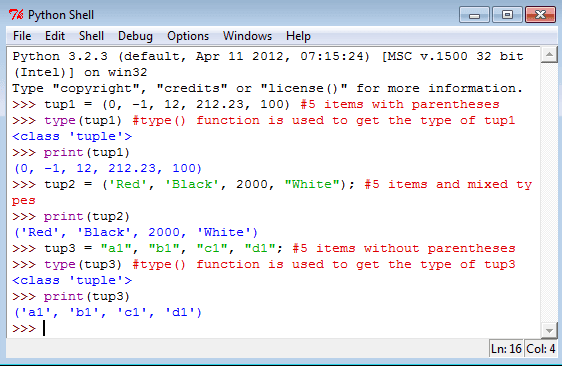To create an empty tuple or create a tuple with single element use the following commands.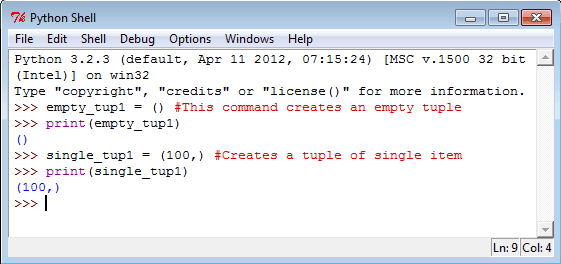Elements of a tuple are indexed like other sequences. The tuple indices start at 0. See the following statements.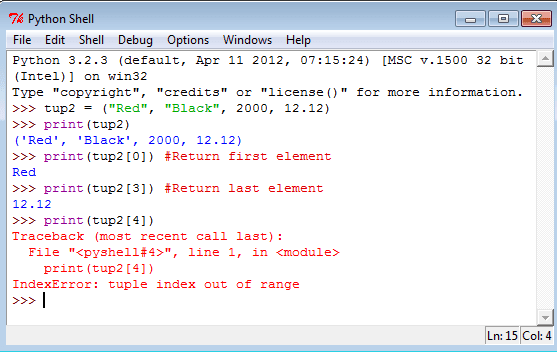Tuples are immutable which means it's items values are unchangeable. See the following statements.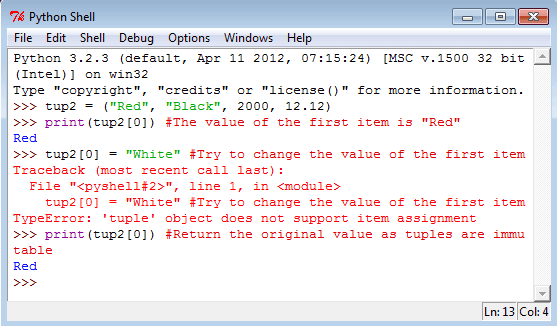## Slicing a tuple

Like other sequences like strings, tuples can be sliced. Slicing a tuple creates a new tuple but it does not change the original tuple.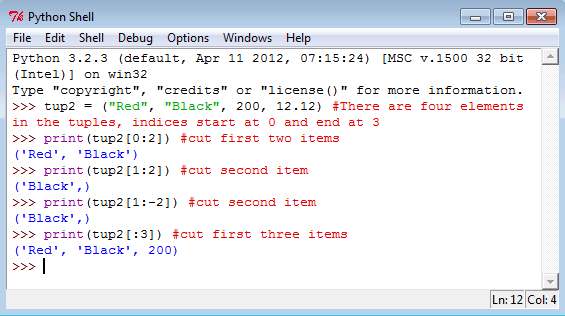## Using + and * operators in Tuples

Use + operator to create a new tuple that is a concatenation of tuples and use * operator to repeat a tuple. See the following statements.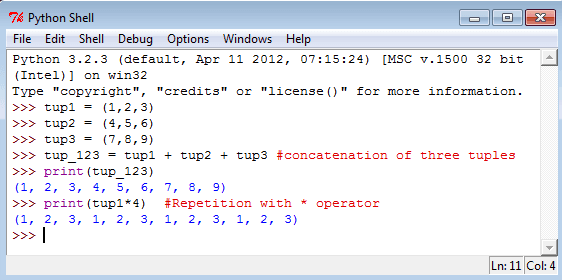## Lists

A list is a container which holds comma-separated values (items or elements) between square brackets where Items or elements need not all have the same type.

## Creating Lists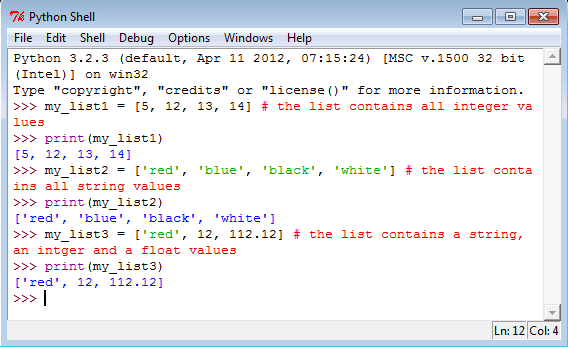A list without any element is called an empty list. See the following statements.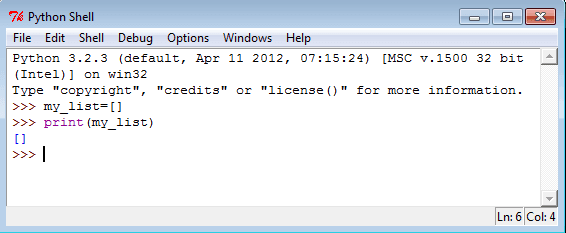## List indices

List indices work the same way as string indices, list indices start at 0. If an index has a positive value it counts from the beginning and similarly it counts backward if the index has a negative value. As positive integers are used to index from the left end and negative integers are used to index from the right end, so every item of a list gives two alternatives indices. Let create a list called color_list with four items.
color_list=["RED", "Blue", "Green", "Black"]

 Item RED Blue Green Black Index (from left) 0 1 2 3 Index (from right) -4 -3 -2 -1

If you give any index value which is out of range then interpreter creates an error message. See the following statements.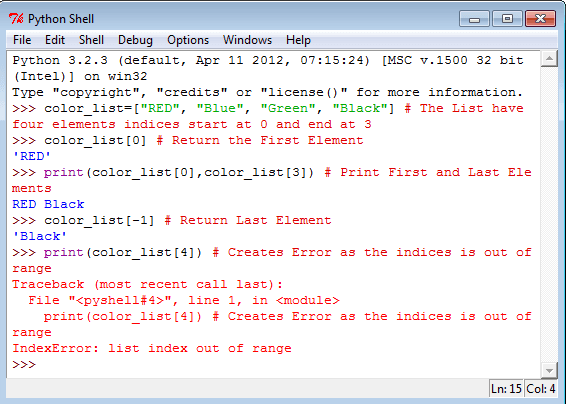## List Slices

Lists can be sliced like strings and other sequences. The syntax of list slices is easy :
sliced_list = List_Name[startIndex:endIndex]
This refers to the items of a list starting at index startIndex and stopping just before index endIndex. The default values for list are 0 (startIndex) and the end (endIndex) of the list. If you omit both indices, the slice makes a copy of the original list. See the following statements.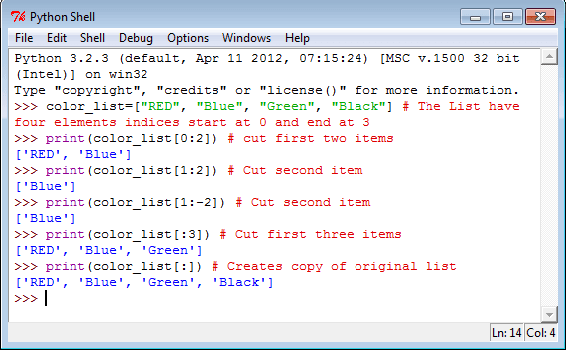## Lists are Mutable

Items in the list are mutable i.e. after creating a list you can change any item in the list. See the following statements.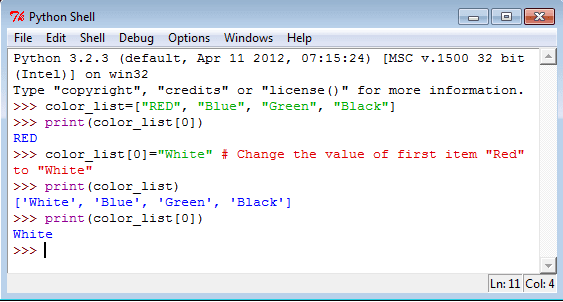## Using + and * operators in List

Use + operator to create a new list that is a concatenation of two lists and use * operator to repeat a list. See the following statements.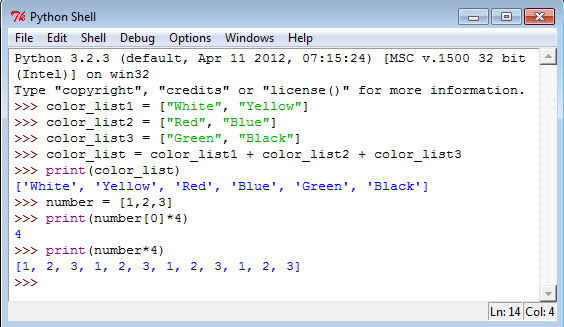## Sets

A set is an unordered collection of unique elements. Basic uses include dealing with set theory (which support mathematical operations like union, intersection, difference, and symmetric difference) or eliminating duplicate entries. See the following statements.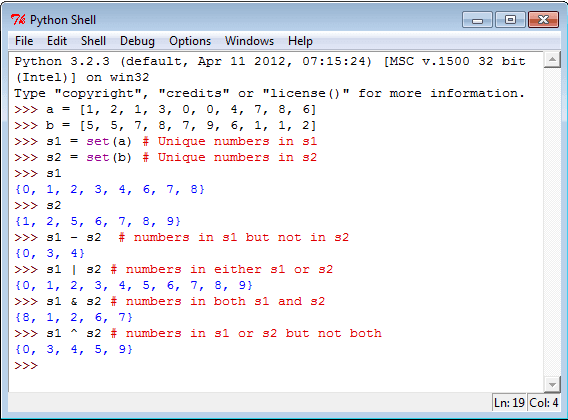## Dictionaries

Python dictionary is a container of the unordered set of objects like lists.The objects are surrounded by curly braces { }. The items in a dictionary are a comma-separated list of key:value pairs where keys and values are Python data type. Each object or value accessed by key and keys are unique in the dictionary. As keys are used for indexing, they must be the immutable type (string, number, or tuple). You can create an empty dictionary using empty curly braces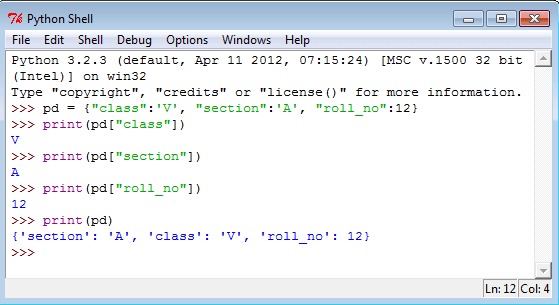## None

This type has a single value. There is a single object with this value. This object is accessed through the built-in name None. It is used to signify the absence of a value in many situations, e.g., it is returned from functions that don't explicitly return anything. Its truth value is false.

Previous: Python Variable
Next: Python Operators

Test your Python skills with w3resource's quiz

﻿

## Python: Tips of the Day

Clamps num within the inclusive range specified by the boundary values x and y:

Example:

```def tips_clamp_num(num,x,y):
return max(min(num, max(x, y)), min(x, y))
print(tips_clamp_num(2, 4, 6))
print(tips_clamp_num(1, -1, -6))
```

Output:

```4
-1
```